Technical Article

# Obtaining Convergence for High-Q XTAL Oscillators

October 30, 2019 by Kevin Aylward

## Learn a method of attaining convergence when simulating very high Q crystal oscillators using Cadence’s Virtuoso Periodic Steady State (PSS) analysis.

When simulating very high Q crystal oscillators using Cadence’s Virtuoso Periodic Steady State (PSS) analysis, it is often very difficult to obtain convergence and thus obtain a simulation of phase noise. This is so despite the options specifically available to improve convergence.

In this article, we'll discuss a method that greatly increases the probability of convergence, and achieves this whilst keeping simulations times short. It is a technique that has proven effective in obtaining convergence where the oscillator is embedded in a hierarchy of hundreds of other circuit blocks.

### Initial Setups

It is important to set simulation options that minimize the work of Spectre in finding a solution such that when PSS does converge; it actually converges to an accurate result. There are some default Spectre settings that are unduly restrictive, and for most designs, are simply an accident waiting to happen—for example, “iabstol”. However, for high Q xtal oscillators, the default reltol accuracy is insufficient.

The suggested starting point is:

• reltol=10e-6
• iabstol=10p
• gmin=10p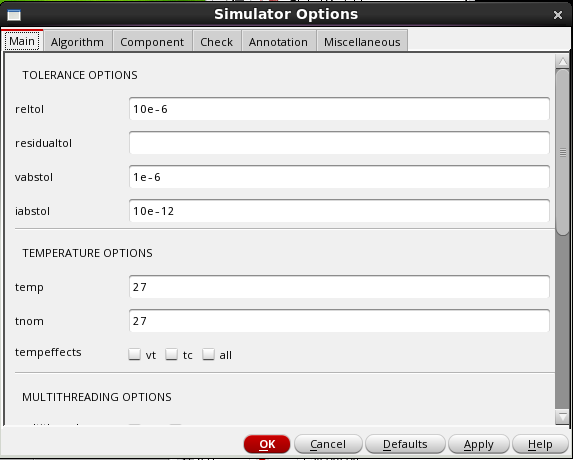Spectre/SPICE defaults are typically 1pA for the current error tolerance and 0.1% for the relative tolerance. SPICE can only converge when variables span a range of no more than about 12 orders of magnitude when using standard double precision arithmetic, so 1pA is very harsh for most circuits. Increasing this value to 100 pA or even 1 nA for large current is sometimes a good idea. However, for reliable accuracy in Phase Noise, the default reltol of 0.1% is nowhere near enough.

A reasonable starting value is 10e-6 but for some circuits this will need to be increased to say, 1e-6.  A tell-tale sign of inaccurate results is the presence of step jumps in the phase noise plots.

### PSS Setup

The PSS form must be set so that a pre trans run “tstab” is always performed. Extensive simulations have shown that, for difficult convergence oscillators, that the options designed to improve convergence, essentially, always fail. That is the detect “steady state” and “calculate initial conditions” should never be engaged.

The suggested starting point is:

• Number of  Harmonics=50
• Accuracy Defaults=Conservative
• Run Transient=YES
• Stop Time=Described below
• Calculate Initial Conditions=Not enabled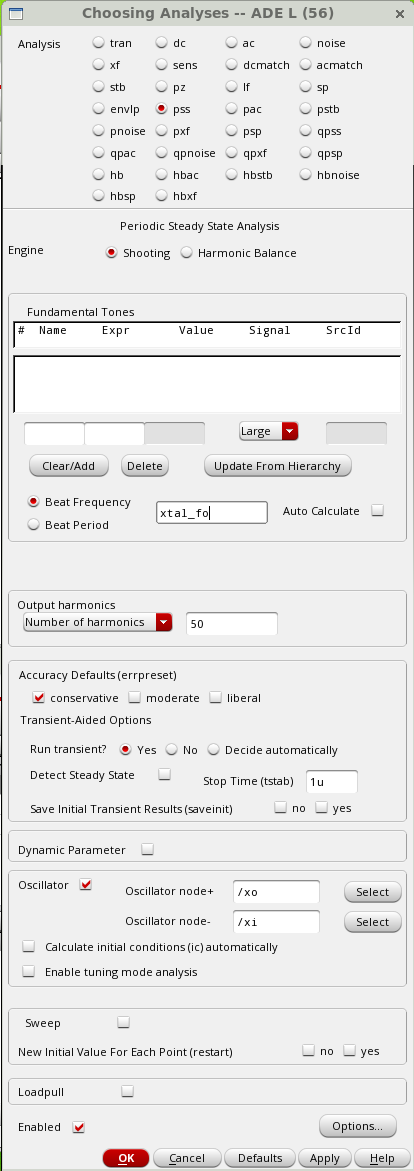The Shooting Method is usually the best method for any oscillator system other than a simple sinewave output. Most oscillator applications require a squaring limiter such that the system is highly nonlinear. Thus a default of 50 harmonics is a good starting point. For particular difficult circuits 100 harmonics may well be required. Again, if the overall Phase Noise plot is not smooth, it indicates that the plot may well be in error. The conservative accuracy setting signals Spectre to actually make the initial 10e-6 setting for retol, even tighter.

Note that, as usual, set the oscillator nodes to the XTAL nodes.

### PNoise Setup

The PNoise setup is relatively standard. For accuracy, set the default maximum sidebands to 50.

In order to reduce simulation time, but still obtain a reasonable smooth plot, a logarithmic plot with 10 points per decade is usually sufficient. Typically the concern is only for phase noise so check the appropriate box.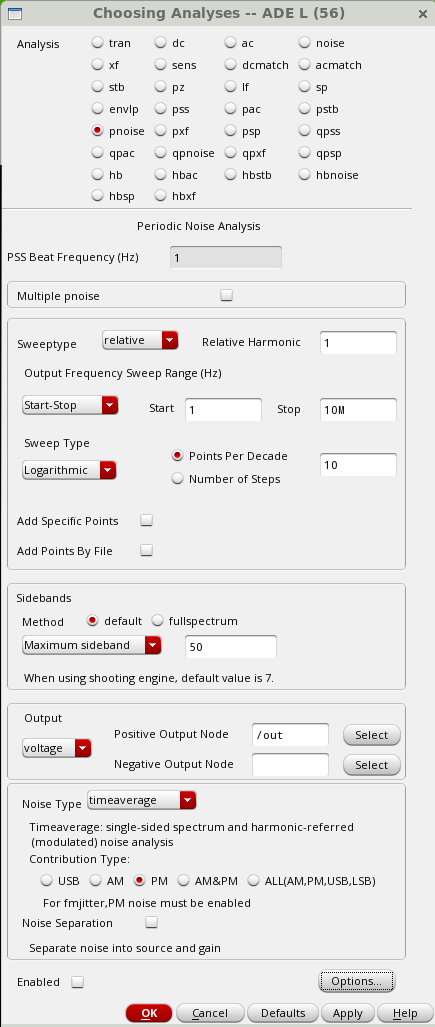### Output/Plotting Setup

To ensure that the oscillator actually works, a Cadence Stability Analysis should be run first.

Unfortunately, Cadence Stability Analysis, at the time of writing this article, has a basic flaw that prevents the loop gain margin and loop phase margin being outputted using its direct plot features. (This is er.. ahhmmm…despite tickets being submitted to their ahmmm… support Dept…)

The Cadence Spectre log will produce the following…

“ WARNING (SPECTRE-16922): Cannot obtain the phase margin and gain margin because the circuit is a positive feedback system and is unstable. This is because the magnitude of loopGain is greater than one at 10.003 MHz when the phase of loopGain crosses zero degree. To make the circuit stable, ensure that the magnitude of loopGain is less than one when the phase of loopGain crosses zero degree.”

So, sure, it’s an oscillator! Just spit out the results anyway Dah!…

So…the output form should be set with a manual script as shown here:

Loop Phase

phaseDegUnwrapped(getData("loopGain" ?result "stb"))

Loop Gain

db(mag(getData("loopGain" ?result "stb")))

Oscilation Frequency

cross(leafValue(phaseDegUnwrapped(getData("loopGain" ?result "stb"))) "0" 1 "either" nil nil nil)

Oscilation Gain

value(leafValue(db(mag(getData("loopGain" ?result "stb")))) cross(leafValue(phaseDegUnwrapped(getData("loopGain" ?result "stb"))) "0" 1 "either" nil nil nil))

Sometimes, depending on the circuit, the phase is shifted in lumps of 360 degs, so the crossing point “0” should be modified appropriately.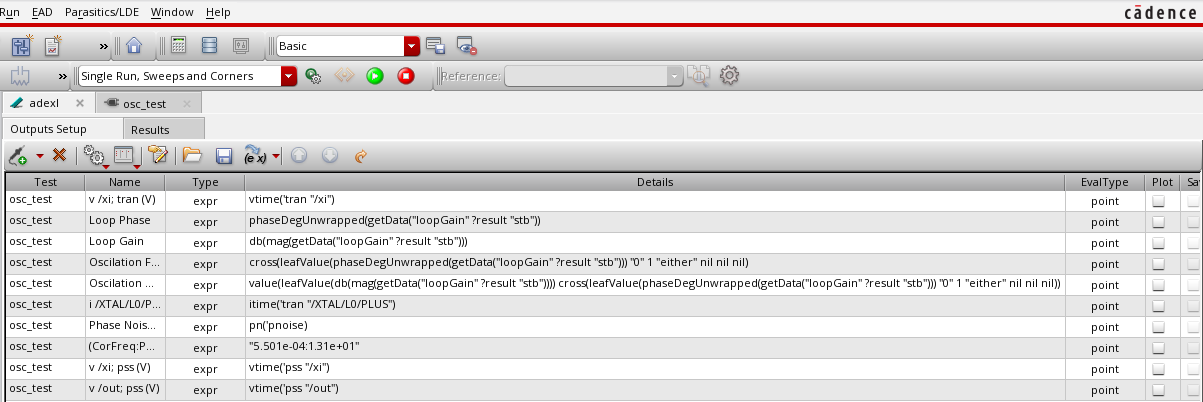### XTAL Model Setup

The schematic for the XTAL should be set up such that the schematic calculates the required XTAL inductance from the c1 of the XTAL and the XTAL frequency.

Thus the inductor should have the following set in its inductance field of its setup form:

1/(pPar("C1")*((2*3.141592654*pPar("FS"))*(2*3.141592654*pPar("FS"))))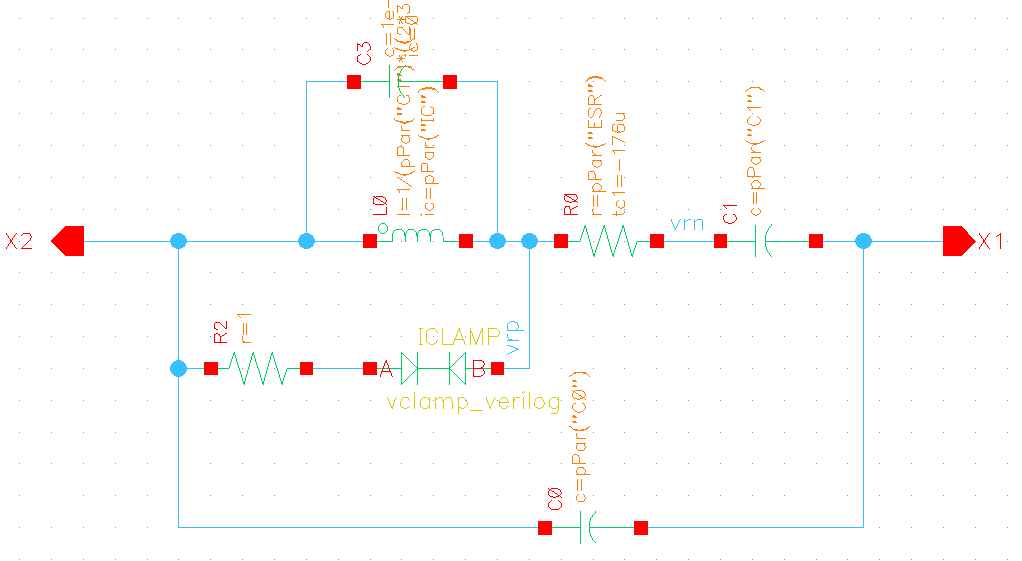The component ICLAMP is a Verilog voltage/current limiter that aids convergence in that high Q XTALS can generate 100kV type numbers, whence SPICE can, during the convergence process, produce even higher voltages. It helps to avoid those “last convergent node=123.8 MV” errors. However, it may not be necessary.

Its code is:

include "constants.vams"

include "disciplines.vams"

module vclamp_verilog(A, B);

inout A;

electrical A;

inout B;

electrical B;

parameter real imax = 0.5 ;

parameter real vmax = 1 ;

parameter real i0 = 1E-18;

analog begin

I(A,B) <+imax*tanh(i0*sinh(100*tanh((40/vmax/100)*V(A,B))));

end

The capacitor across the inductor is a dummy capacitor of very small value, typically 1e-20F. It is required as a convenient method to force the initial voltage across the inductor to 0V. This node voltage setting is part of this convergence technique.

### Convergence Method

The problem for convergence of high Q XTALS is that Spectre has difficulty in convergence simply because the Q is high. For the same circuit, but with a lower Q, it usually has little problem. Thus, the method is to solve for a low-Q circuit and use that result to aid in the solution at full Q.

The key principle is that a low Q XTAL will reach its steady state value much quicker than a high Q XTAL. That is, if the XTAL is “De-Qed” by a factor of 100, then the simulation will be 100 times quicker to settle.

The Q of an XTAL oscillator is determined by the C1 (series resistance) of the XTAL. However, the steady state current in the inductor of the XTAL is independent of C1. Thus the low-Q inductor current may be used as the initial current for the full Q XTAL.

So, the principle of the method is to initialize the inductor current with a current close to what it would be in steady state, with that current determined by first running a low Q version of the circuit.

A convenient way to set up the simulation is to introduce a variable—say, QR—that multiplies the C1 so that QR is first set to say, 100 for a low Q run, then set to 1 for the full Q run. For example: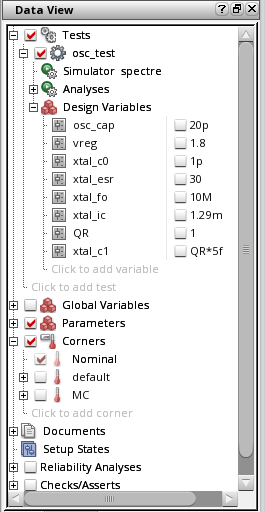### Example Schematic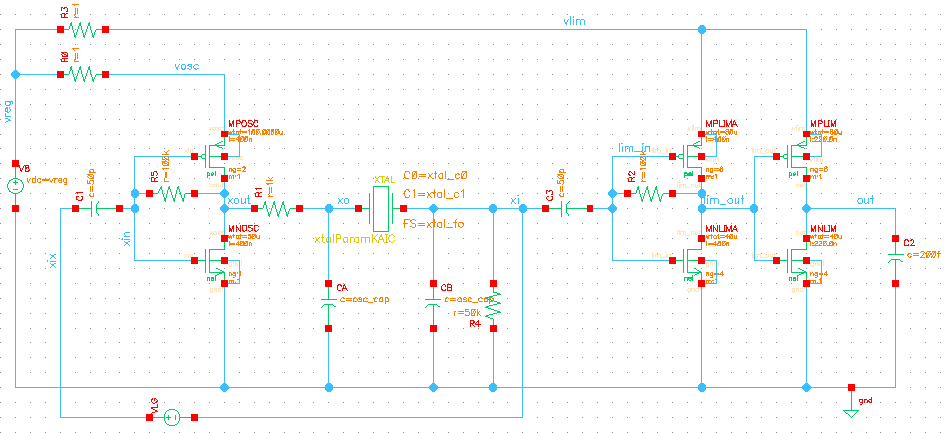### Example Waveforms

The top graph shows the signal voltages at X1 for both the low Q and High Q runs. The bottom graph shows the inductor current for both the low Q and high Q runs.

It can be seen that the value determined from the low Q configuration, allows the high Q configuration to start, essentially, immediately.

This allows PSS a much better starting condition so that it is more likely to converge. In this particular case, the PSS tstab time was only set to 1us. For difficult cases, it will need to be determined empirically.

#### TRAN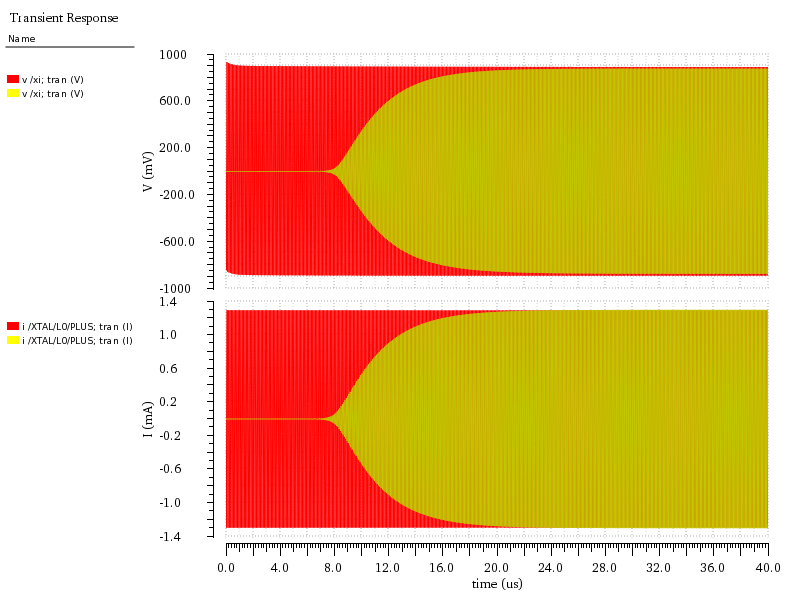#### PSSR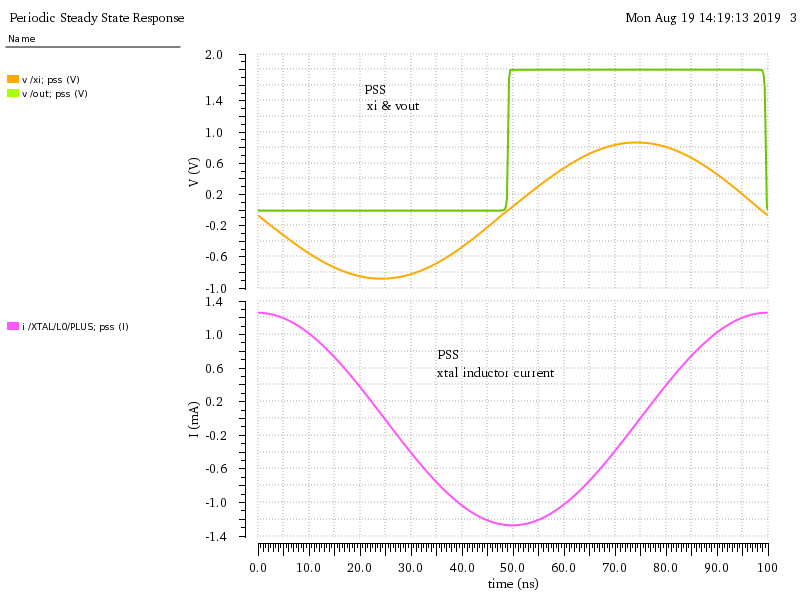#### Phase Noise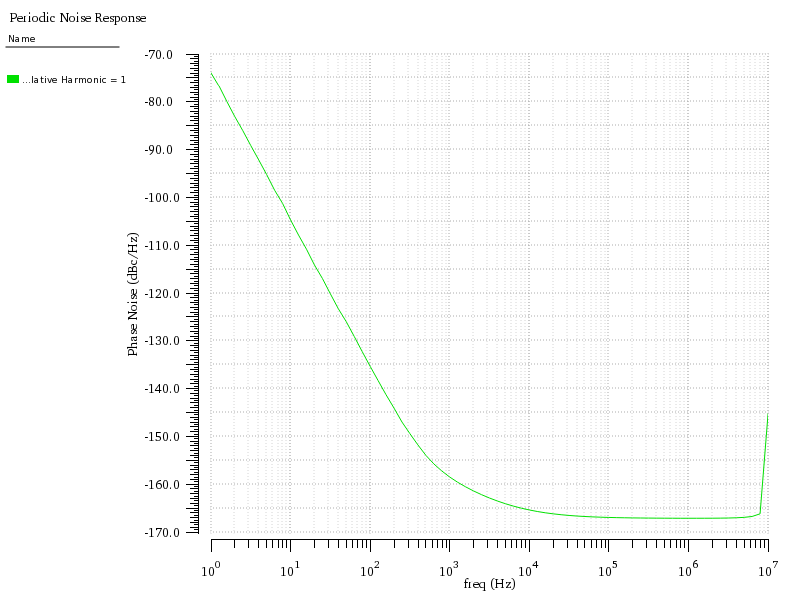What are your tips and tricks for working in simulation software? Share your experience in the comments below.